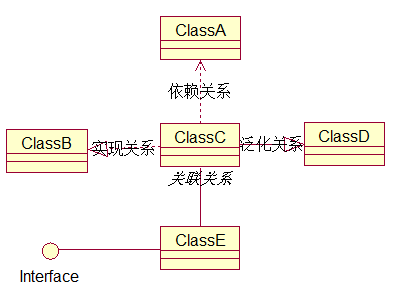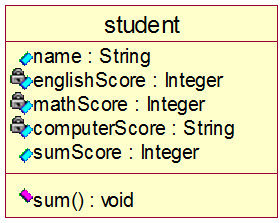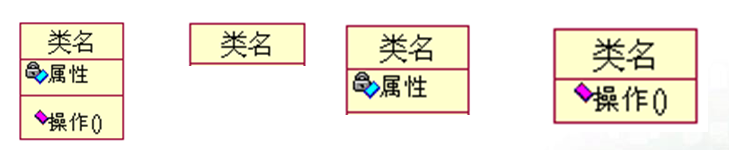### 2.种类元素（类和接口）

4种关系（依赖关系、实现关系、泛化关系和关联关系）。### 3.类的概念与表示符号

```class student{ //定义学生类
public string name;
private int englishScore;
private int mathScore;
private int computerScore;
public int sumScore;

public student(string x, int y, int  z, int k){
name = x;
englishScore = y;
mathScore = z;
computerScore =k;
}

public void sum(){
sumScore = englishScore + mathScore + computerScore;
}
}```